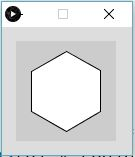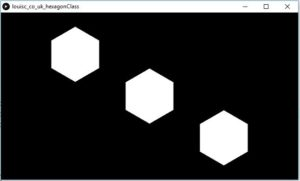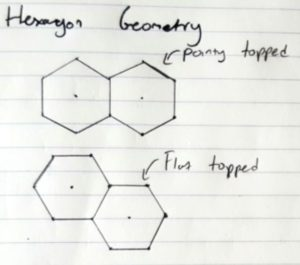# Tinkering in Processing – Drawing a Hexagonal Grid

/, Projects, Tinkering/Tinkering in Processing – Drawing a Hexagonal Grid

## Tinkering in Processing – Drawing a Hexagonal Grid

My friend Tine (Her cool Artwork here) has a new gig; an interactive swing set for display in the art exhibition at SIGGRAPH 2016, probably the largest computer graphics and interactive techniques conference. One of the reasons I feel Tine and I work so well together is we both appreciate the benefits of an iterative design process when molding technology to artistic ideas. Mess with ideas in the prototype stage, keep things as modular and flexible as possible and always plan for future expansion!

So, I have never built an interactive computer visualization before, but i have dabbled with processing and have a rough idea of what will be involved. From our initial brainstorming session we were keen on a honeycomb grid structure which formed a good starting point for coding.

This is a super quick processing animation made by randomly filling boxes to test the code described below:

View post on imgur.com

## Drawing a Hexagon in Processing

Next was a trip down memory lane, some basic trig! The idea here is, in order to create a hexagon, we can calculate the position (x, y) of the 6 vertices which form the “pointy bits” in our 2D space then connect them together to form our shape.

Starting from what we define as the center of the hexagon, the first known value we can calculate is the angle. Since processing works in radians rather than degrees, we will make our calculations as such. (For anybody reading who is not used to working in radians, the concept may seem alien but it is very straight forward, promise! Google for 10 mins, you’ll see!)

We know a full circle forms and angle of 2? radians (360 degrees), so we simply divide this by 6 to calculate the angle our triangular calculation will form, essentially giving an angle of 2?/6 (60 degrees). In code we can then cumulatively add this angle in a for loop until we reach all the way around the hexagon. In code this looks a little like this:

float centx = 50;
float centy = 50;
float angle = TWO_PI / 6;

beginShape();
for (float a = PI/6; a < TWO_PI; a += angle) {
float vx = centx + cos(a) * radius;
float vy = centy + sin(a) * radius;
vertex(vx, vy);
}
endShape(CLOSE);


This results in an output of:The beginShape() and endShape() commands result in the creation of a shape object from the set of vertices drawn within. By including the CLOSE command processing will draw the final line back to the first vertex closing the shape.

## An Object Oriented Approach

Alright so we drew our hexagon with defined center points and radius, great! Now we need a grid of these, and better yet, since we plan to animate and gameify this grid we also need to store certain properties for each one.

### A Hexagon Class

So, we have the code which draws a Hexagon along with the variables required to define its position and radius. For now the only additional property we will code in is a colour. So my initial draft for a class looks something like this:

class Hexagon {
float centx;
float centy;
float angle = TWO_PI / 6;
boolean fill = false;
color c;

//Our Constructor takes the center coordinates along with a value for radius
Hexagon( float x, float y, float r )
{
centx = x;
centy = y;
}

//The draw function will define the fill values and calculate the coordinates
void draw() {
if(fill)
fill(c);
else
noFill();

beginShape();
for (float a = PI/6; a < TWO_PI; a += angle) {
float sx = centx + cos(a) * radius;
float sy = centy + sin(a) * radius;
vertex(sx, sy);
}
endShape(CLOSE);
}

//The following are all simply utility functions for setting parameters
float centx()
{
return centx;
}

float centy()
{
return centy;
}

color getColour()
{
return c;
}

void setFillColour(color col)
{
fill = true;
c = col;
}

void setNoFill(boolean yesno)
{
fill = yesno;
}
}


A quick test of our new class:

//Lets define our array of hexagon objects globally
Hexagon[] hexagons;

void setup()
{
size(640, 360, P3D);
//fullScreen(P2D);
background(0);
smooth();
hexagons=new Hexagon;
//Manually define each new hexagon object's coordinates and radius
hexagons = new Hexagon(width/4, height/4, radius);
hexagons = new Hexagon(width/2, height/2, radius);
hexagons = new Hexagon(3*width/4, 3*height/4, radius);
}

void draw()
{
for( int i=0; i<3; i++ )
{
hexagons[i].draw(); //Cycle though our short array and call the draw function
}
}


Resulting in:Great! We can now draw hexagons on demand!

# Generating a Hexagonal Grid

In order to easily reference each hexagon on our grid with a column and row style system we will generate a class simply named “HexGrid”. This will be responsible for drawing and storing the hexagon objects which form each cell, as well as allowing us to retrieve any given hexagon by provide a column and row number.

First a simple bit of geometry math. In order to generate a grid of hexagons we must calculate the x and y offsets which allow them to fit together in the honeycomb style arrangement. First we need to decide on the standard orientation of our hexagons, as this site suggests we can have “pointy” or “flat” topped grid.The height is easy to calculate, since the radius is measures to the points, the height is defined as twice the radius:The width of a hexagon is defined as:which simply becomes:Since horizontally each hexagon will be touching, this defines our x displacement. For the y displacement we will essentially be sitting the next row 3/4 away from its previous row (with the start offset by 1/2 a width) so that the “pointy” top fits within corresponding point left by the two meeting hexagons above. So finally giving:(A great resource used for guidance in deciding how to go about this was: http://www.redblobgames.com/grids/hexagons/. There is some great analysis and live examples of the math, grid coordinate referencing and pseudo code implementations on hex grids!)

Creating the grid is now a pretty straight forward task of two nested for loops, as such the final HexGrid class is shown below. I have added comments in the key areas:

class HexGrid {
Hexagon[][] grid; //Our 2D storage array of Hexagon Objects
int cols, rows;

//Class Constructor required the grid size and cell radius
HexGrid(int nocol, int norow, int rad)
{
//Define our grid parameters
cols = nocol;
rows = norow;

//2D Matrix of Hexagon Objects
grid=new Hexagon[cols][rows];

//Lets assign the inital x,y coordinates outside the loop

//These two nested for loops will cycle all the columns in each row
//and calculate the coordinates for the hexagon cells, generating the
//class object and storing it in the 2D array.
for( int i=0; i < rows ; i++ ){
for( int j=0; j < cols; j++)
{
grid[j][i] = new Hexagon(x, y, radius);
x+=radius*sqrt(3); //Calculate the x offset for the next column
}
y+=(radius*3)/2; //Calculate the y offset for the next row
if((i+1)%2==0)
else
}
}

//This function will redraw the entire table by calling the draw on each
//hexagonal cell object
void draw()
{
for( int i=0; i < rows ; i++ ){
for( int j=0; j < cols; j++)
{
grid[j][i].draw();
}
}
}

//This function will return the hexagonal cell object given its column and row
Hexagon getHex(int col, int row)
{
return grid[col][row];
}

}


Now we can have some fun by generating a grid and randomly filling it with colours 🙂
Here is the code, once again comments should describe whats going on:

/*
Louis Christodoulou - Hexagonal Grid Test
May 2016
*/

HexGrid g;

void setup()
{
size(1920, 1080);
smooth();
background(40);

//Define some of our grid parameters

//Generate our grid object by calling the constructor
g = new HexGrid(nwide, nhigh, rad);
//Draw our newly generated grid
g.draw();

frameRate(60);
}

void draw()
{
//Since our grid cells now have a state, we can redraw our grid without loosing changes
g.draw();

//Each time draw loops we select 5 random hexagons in our grid and assign a
//random colour from the pallet
for(int i=0; i < 5 ; i++)
{
Hexagon selected = g.getHex(int(random(nwide)), int(random(nhigh)));
selected.setFillColour(mainpallet[int(random(2))]);
}
}


Thanks for reading and please do pop a comment below if you have questions, a better way of doing things, i have made a mistake or sharing your work which is similar!

By |2016-11-05T11:17:47+00:00June 19th, 2016|Kinetic Storyteller, Projects, Tinkering|6 CommentsA PhD in Electronic Engineering. A love for photography (www.islou.co.uk). An interest in tinkering, electronics and design. (www.louisc.co.uk).

1. […] Home/Kinetic Storyteller, Projects, Tinkering/Drawing Arcs and Text with the Geomerative Processing Library Previous […]

2.makio135 July 7, 2016 at 8:54 pm - Reply

Hey, you should have a look at the PShape class, create one global instance with the hexagon shape, and draw it from the center ( with shapeMode(CENTER) ) for every positions in the grid instead of calculating each hexagons on each frame 😉

•louis July 7, 2016 at 9:33 pm - Reply

Thanks Makio, just checked it out on the processing site! You’re totally right! That would be a nicer way of doing it, suppose you would create a PShape object, say named hexagon then call the beginShape() method.

Looking at the reference docs I believe perhaps the code above may be generating a PShape object anyhow (behind the scenes) but with no reference so once its drawn its just deleted ?

Thanks for the heads up! Newbe here, so much to learn!

3.S.B. April 16, 2017 at 2:44 pm - Reply

Let’s say you wanted to make a method that returns the 0-6 hexagons surrounding a hexagon inside a 2D array. How would you do this?

•louis April 30, 2017 at 12:36 pm - Reply

Hi S.B., saw your comment but was a bit of a crazy period. Had a bit of free time and thought I’d have a quick crack at it. Not sure if its still useful….

There are probably far more fancy ways of doing this but i settled for a simple “ArrayList getNeighbors(int tcol, int trow)” function added to the grid function.

Just on the back of an envelope I figured which translations (to the grid reference) make the surrounding cells:
int col_trans_o[] = {0, 1, 1, 1, 0, -1};
int col_trans_e[] = {-1, 0, 1, 0, -1, -1};
int row_trans[] = {-1, -1, 0, +1, +1, 0};

Then I scan through these, and if they are valid (I.e. exist on the grid) then i add them to the Array and return them!

// Find our neighbors and append to a list
for(int i=0; i<6; i++){
int col;
int row = trow + row_trans[i]; //Translate Row
if( row < 0 || row >= rows )
continue;
// Check if row is even or odd
if( row%2 == 0 ) //Translate Col
col = tcol + col_trans_e[i];
else
col = tcol + col_trans_o[i];
if( col < 0 || col >= cols )
continue;
•